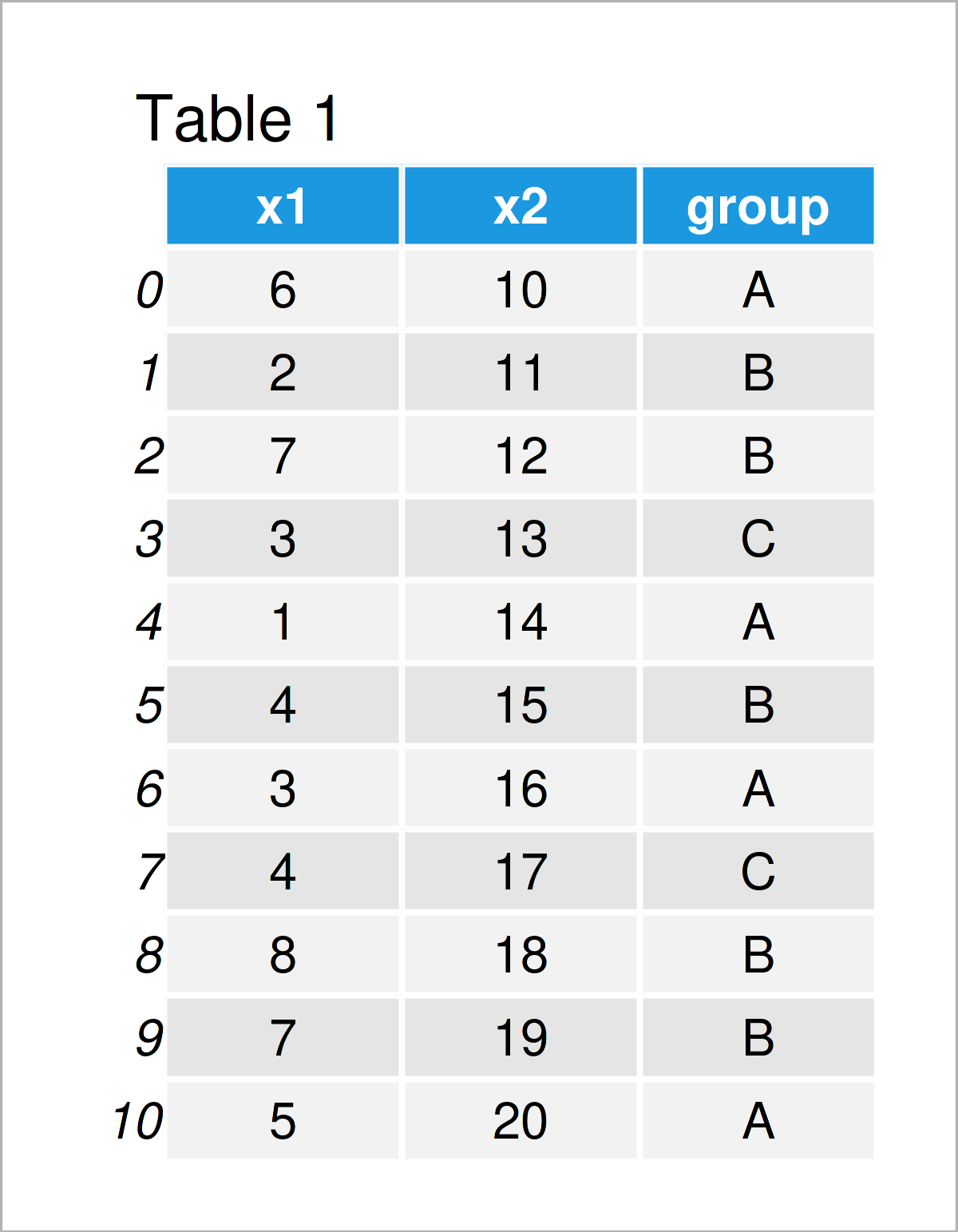# Quantile in Python (4 Examples)

In this tutorial you’ll learn how to get quantiles of a list or a pandas DataFrame column in Python programming.

The tutorial contains these contents:

Let’s get started:

## Example 1: Quantiles of List Object

In this example, I’ll show how to calculate the quantiles of a list object in Python.

Let’s first create an example list:

```my_list = [8, 4, 4, 3, 2, 4, 1, 3, 5, 2, 1, 3, 7]             # Create example list
print(my_list)                                                # Print example list
# [8, 4, 4, 3, 2, 4, 1, 3, 5, 2, 1, 3, 7]```

Furthermore, we have to import the NumPy library:

`import numpy as np                                            # Load NumPy library`

Now, we can use the quantile function of the NumPy package to create different types of quantiles in Python.

The following syntax returns the quartiles of our list object. Note that we are using the arange function within the quantile function to specify the sequence of quantiles to compute. Since we want to find the quartiles of our list, we have to specify a sequence containing the values 0.25, 0.5, and 0.75:

```print(np.quantile(my_list, q = np.arange(0.25, 1, 0.25)))     # Get quartiles of list
# [2. 3. 4.]```

As you can see, the values 2, 3, and 4 have been printed (i.e. the first second and third quartile).

We can modify the sequence within the quantile function to get other kinds of quantiles. The following Python code prints the deciles…

```print(np.quantile(my_list, q = np.arange(0.1, 1, 0.1)))       # Get deciles of list
# [1.2 2.  2.6 3.  3.  4.  4.  4.6 6.6]```

…and the Python syntax below returns the percentiles of our list:

```print(np.quantile(my_list, q = np.arange(0.01, 1, 0.01)))     # Get percentiles of list
# [1.   1.   1.   1.   1.   1.   1.   1.   1.08 1.2  1.32 1.44 1.56 1.68
#  1.8  1.92 2.   2.   2.   2.   2.   2.   2.   2.   2.   2.12 2.24 2.36
#  2.48 2.6  2.72 2.84 2.96 3.   3.   3.   3.   3.   3.   3.   3.   3.
#  3.   3.   3.   3.   3.   3.   3.   3.   3.12 3.24 3.36 3.48 3.6  3.72
#  3.84 3.96 4.   4.   4.   4.   4.   4.   4.   4.   4.   4.   4.   4.
#  4.   4.   4.   4.   4.   4.12 4.24 4.36 4.48 4.6  4.72 4.84 4.96 5.16
#  5.4  5.64 5.88 6.12 6.36 6.6  6.84 7.04 7.16 7.28 7.4  7.52 7.64 7.76
#  7.88]```

Note that we could use the same logic to return other kinds of quantile values such as terciles, quintiles, sextiles, septiles, octiles, duodeciles, vigintiles, and permilles.

## Example 2: Quantiles of One Particular Column in pandas DataFrame

In this example, I’ll demonstrate how to get the quantiles of a specific column in a pandas DataFrame.

First, we have to import the pandas library:

`import pandas as pd                                           # Load pandas library`

Also, we have to create an exemplifying pandas DataFrame:

```data = pd.DataFrame({'x1':[6, 2, 7, 3, 1, 4, 3, 4, 8, 7, 5],  # Create pandas DataFrame
'x2':range(10, 21),
'group':['A', 'B', 'B', 'C', 'A', 'B', 'A', 'C', 'B', 'B', 'A']})
print(data)                                                   # Print pandas DataFrame```After running the previous code the pandas DataFrame shown in Table 1 has been created. It contains the two float variables x1 and x2 as well as a group indicator.

If we want to find the quartiles of a certain variable in our data set (i.e. x1), we can use the following Python code:

```print(data['x1'].quantile(np.arange(0.25, 1, 0.25)))          # Get quartiles of one column
# 0.25    3.0
# 0.50    4.0
# 0.75    6.5
# Name: x1, dtype: float64```

Similar to that, we can calculate the deciles…

```print(data['x1'].quantile(np.arange(0.1, 1, 0.1)))            # Get deciles of one column
# 0.1    2.0
# 0.2    3.0
# 0.3    3.0
# 0.4    4.0
# 0.5    4.0
# 0.6    5.0
# 0.7    6.0
# 0.8    7.0
# 0.9    7.0
# Name: x1, dtype: float64```

…and the percentiles of a pandas DataFrame column:

```print(data['x1'].quantile(np.arange(0.01, 1, 0.01)))          # Get percentiles of one column
# 0.01    1.1
# 0.02    1.2
# 0.03    1.3
# 0.04    1.4
# 0.05    1.5
...
# 0.95    7.5
# 0.96    7.6
# 0.97    7.7
# 0.98    7.8
# 0.99    7.9
# Name: x1, Length: 99, dtype: float64```

## Example 3: Quantiles of All Columns in pandas DataFrame

In the previous example, I have illustrated how to return the quantiles of a single pandas DataFrame column.

In this section, I’ll explain how to return the quantiles of all pandas DataFrame columns in one single call of the quantile function.

For this task, we can use the Python code below:

```print(data.quantile(np.arange(0.25, 1, 0.25)))                # Get quartiles of all columns
#        x1    x2
# 0.25  3.0  12.5
# 0.50  4.0  15.0
# 0.75  6.5  17.5```

The previous output shows the quartiles for each column in our data set.

## Example 4: Quantiles by Group in pandas DataFrame

In Example 4, I’ll demonstrate how to calculate quantile values by group.

To accomplish this, we have to separate our data using the groupby function as shown below. Note that we have specified only one value (i.e. 0.25) within the quantile function to return only the first quartile by group.

```print(data.groupby('group').quantile(0.25))                   # Get first quartiles by group
#          x1    x2
# group
# A      2.50  13.0
# B      4.00  12.0
# C      3.25  14.0```

## Video, Further Resources & Summary

Would you like to know more about the computation of quantiles of a list and a pandas DataFrame column? Then I can recommend having a look at the following video on my YouTube channel. In the video, I’m explaining the contents of this article.

In addition, you could read the related articles on this website. A selection of tutorials is listed here.

Summary: In this Python programming tutorial you have learned how to calculate quantiles. If you have any further questions, let me know in the comments.

Subscribe to the Statistics Globe Newsletter